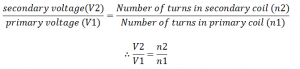# Transformer and its structure

• It is a device that converts high voltage AC to low voltage AC of the same frequency and vice versa.
• It works on the principle of mutual induction, i.e., whenever the current (magnetic flux) through a coil changes, an e.m.f. is induced in the neighbouring coil.
• According to this principle, when the electric current in a closed circuit (primary coil) changes, the changing current (AC) creates a changing magnetic field.
• A second circuit (secondary coil) in reach of this magnetic field will experience this change in the magnetic field as a change in its related magnetic flux.
• Therefore, an electromotive force is set up in the second circuit (secondary coil) called an induced e.m.f (or transformer e.m.f).
• If the two ends of the secondary coil are connected through an electrical load, current will flow.
• A transformer is widely used in electric and electronic devices, such as the radio, TV, trolley bus, factories, computers, etc.

Structure of transformer

• A transformer consists of two separate coils winding on a laminated iron core.
• The two coils are called primary coil and secondary coil.
• The coil of the transformer in which AC voltage is given is called primary coil and the coil from which AC voltage is taken is called secondary coil.
• The iron, core, when used in a single block, develops eddy current, and the core is heated up.
• The heat so produced damages the internal insulation besides causing loss of energy.
• In order to minimize the heating effect, thin insulated metal sheets are adjusted to make the core of a transformer.• These iron sheets are coated with insulating materials like varnish or shellac.
• This process is called lamination.
• The core of a transformer is laminated to minimize energy loss due to the production of eddy current.

Mutual induction

• A changing current (AC) in the first circuit (the primary) creates a changing magnetic field.
• This changing magnetic field induces a voltage change in the second circuit (the secondary).
• This effect is called mutual induction.

Primary voltage (V1) and Secondary voltage (V2)

• The input voltage of a transformer is called primary voltage (V1) and the output voltage is called secondary voltage (V2).
• The number of turns in the secondary coil(n2) can be calculated by the following relation• By the appropriate selection of the number of turns, a transformer thus allows an alternating voltage to be stepped up or stepped down.
• Two laws on which a transformer is based on:
1. In a transformer, the input power (I1V1) is equal to the output power (I2V2), where I1= input current, V1 =input voltage, I2 =output current and V2 =output voltage.
2. The magnitude of the induced e.m.f is directly proportional to the ratio of the number of turns in the secondary coil to the number of turns in the primary coil.

References: